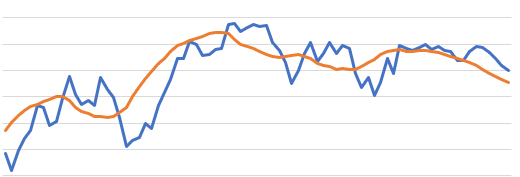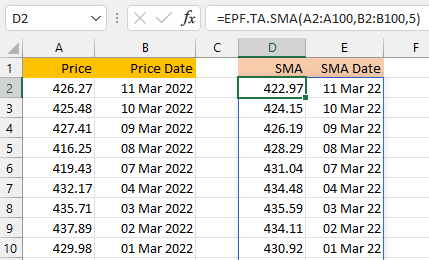# Technical Indicators: Simple Moving AverageThe Simple Moving Average (SMA) indicator is the average price over a lookback window.

The SMA is used to smooth price data and can be used to help determine the direction of a trend. If the SMA is moving up then the trend is moving up and vice-versa.

A price crossing the SMA is often used as a trading signal. For example if the price moves above the SMA then this may signal a long trade and when the price moves below the SMA this may signal a short trade.

You can also calculate more than one SMA and use the points where the SMAs cross as another entry signal.

The Excel formula for calculating the Simple Moving Average is:

` =EPF.TA.SMA(prices, dates, lookbackWindow)`

The input parameters required are:

Parameter Description
Prices An Excel range of the prices.
Dates An Excel range of the dates.
Lookback Window The number of periods in the lookback window.

The range of prices and dates must correspond and be of the same length, for example the first date in the date range must correspond to the first price in the price range.

The output columns are:

Output Column Description
SMA The simple moving average.
Date The date that the simple moving average corresponds to.

Example

"Calculate the SMA with a 5 day lookback window"

In this example you can see the price/date data in columns A and B respectively. The SMA formula is in cell D2 and references the price (A2:A100) and date (B2:B100) ranges as well as the 5 period lookback:

` =EPF.TA.SMA(A2:A100,B2:B100,5)`

The formula calculates the SMA and returns the SMA and corresponding dates to columns D and E respectively: